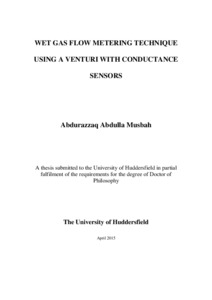Musbah, Abdurazzaq (2015) Wet Gas Flow Metering Technique Using a Venturi With Conductance Sensors. Doctoral thesis, University of Huddersfield.
Abstract

Wet gas metering is becoming an increasingly important problem to the oil and gas industry. Much research has been done to measure the water and gas flow rates of two-phase flows using a Venturi meter. The Venturi meter is a favoured device for metering of unprocessed wet natural gas production flows. In this thesis, various combinations of techniques have been employed in annular gas-liquid two phase flows to measure the flow parameters (e.g. liquid film thickness, gas volume fraction and gas and water flow rates). One of the most useful techniques which was used and which has proven attractive for many previous multiphase flow applications is the electrical conductance technique used in conjunction with the Venturi.
In this thesis, research has been done on designing a novel wet gas flow metering technique, which combines a Venturi with conductance sensors at the inlet and throat to measure the gas and the water flow rates and gas mass flow rate in vertical annular (wet gas) flows. Two ring conductance sensors at the inlet of the Venturi were used to measure the film velocity by cross correlation, one of the inlet ring conductance sensors was used to measure the film thickness and the inlet gas volume fraction. A ring conductance sensor at the throat was used to measure the gas volume fraction at the Venturi throat. A digital level sensor was also used to measure the film thickness (and the gas volume fraction) at the inlet of the Venturi. The reason for measuring the film thickness, the film velocity, the gas volume fraction at the inlet and the throat of the Venturi was to determine the gas and water flow rate and the gas mass flow rate in annular wet gas two phase flow using a variety of mathematical models of Venturis.
This work included both static ‘bench’ and flow loop experiments. In the flow loop experiments, which were limited to air-water two-phase flow, the test section included a Venturi meter with a 50mm inlet diameter with conductance sensors at inlet and the throat. Reference measurements of the water and air were made with a turbine flow meter and a variable area flow meter respectively. A Honeywell DP cell sensor was used to measure the differential pressure between the inlet and the throat of the Venturi.
A NI USB 6009 data acquisition device was used to integrate the system measurements and to control the operation of the overall Venturi system. A program was created using Labview software to read the input signals from the throat conductance ring sensor; the inlet conductance ring sensors; the digital level sensor; the DP cell sensor; the variable area flow meter and the turbine meter. From these measurements the gas volume fraction at the Venturi inlet and the throat, the differential pressure between the inlet and the throat of the Venturi, the film thickness and the film velocity at the Venturi inlet were calculated and were used with appropriate mathematical models to calculate the gas volumetric rate; gas mass flow rate; and the water mass and volumetric flow rates. Reference values of the gas a water flow rates were also calculated from the turbine meter and variable area flow meter outputs.

Information
Library
DocumentsPreview
Musbah_Final_Thesis.docx.pdf - Accepted Version